# Ordering Numbers to 100

Ordering Numbers to 100• To put numbers in order from smallest to largest, look at the digits individually.
• The order of digits from smallest to largest is: 0, 1, 2, 3, 4, 5, 6, 7, 8 and then 9.
• First we can look at numbers with only one digit.
• 4 is the only number with one digit and so it is the smallest. It does not have any tens.
• We cross off each number as we write it.
• Next we look at two digit numbers with a tens digit of ‘1’.
• 17 is the only digit with one ten, so it is next.
• There are two numbers with a digit of ‘2’ in the tens: 21 and 28.
• Since these numbers both have a ‘2’ in the tens, we look at the units (or ones) column to decide.
• ‘1’ comes before ‘8’ in our list of digits and so, 21 is smaller than 28.
• We move on to the next digit in the tens column which is ‘3’.
• Only 36 has a ‘3’ as its tens digit, so it is the next largest.
• No numbers in this list have a tens digit of ‘4’, so we then look at the numbers with a tens digit of ‘5’.
• We have 58 and 59. ‘8’ comes before ‘9’ in our list of digits and so, 58 is smaller than 59.
• Finally, the largest number is 63 as it has a ‘6’ in its tens column. It has more tens than the other numbers.

Put numbers in order by looking at each place value column from left to right.

If these digits are the same, look at the next digit on the right.• To order a set of numbers from least to greatest, order the digits in each place value column from left to right.
• The order of digits from smallest to largest is: 0, 1, 2, 3, 4, 5, 6, 7, 8 and 9.
• Both 2 and 7 have no tens. We order these numbers by looking at the order of the digits above.
• 2 is smaller than 7.
• We next look at the numbers that contain a ‘1’ in the tens column: 11, 12 and 14.
• Looking at the units digit ‘1’ comes before ‘2’ which comes before ‘4’ and so we have 11, 12 and then 14.
• Next we look at the numbers with a ‘2’ in the tens column: 22, 25 and 29.
• ‘2’ comes before ‘5’ which comes before ‘9’ and so the order of these numbers is 22, 25 and 29.Supporting Lessons# How to Order Numbers to 100 from Least to Greatest

In this lesson we are ordering whole numbers between 1 and 100.

Here are the steps to order all whole numbers from smallest to largest:

• If the whole number has more digits than another, then it is larger.
• For whole numbers with the same number of digits, the larger number will have the larger first digit.
• If this digit is the same then compare the next digit to the right of this.

The order of the digits from smallest to largest is:

0, 1, 2, 3, 4, 5, 6, 7, 8 and 9.

In this lesson we are specifically looking at numbers with up to two digits and so we compare the tens place value column and if this digit is the same, then we compare the digits in the units column.

Below is an example of putting numbers in order.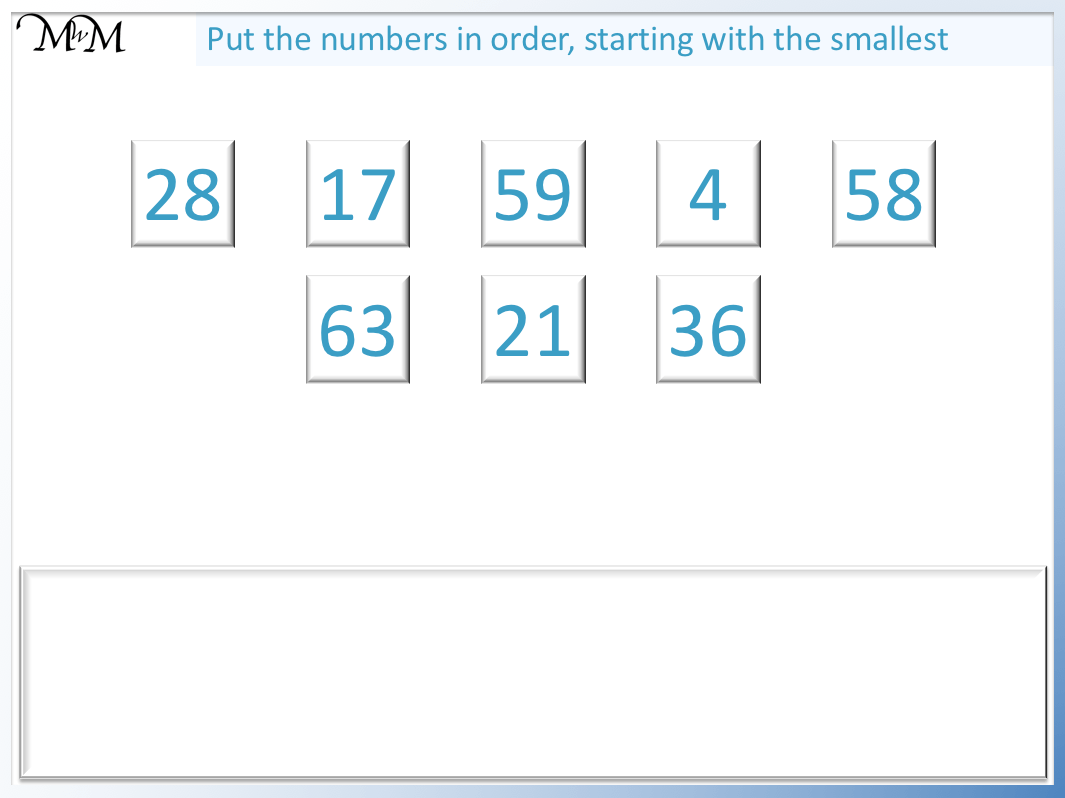We will use the steps above to begin ordering these numbers.

There is only one single-digit number, which is 4.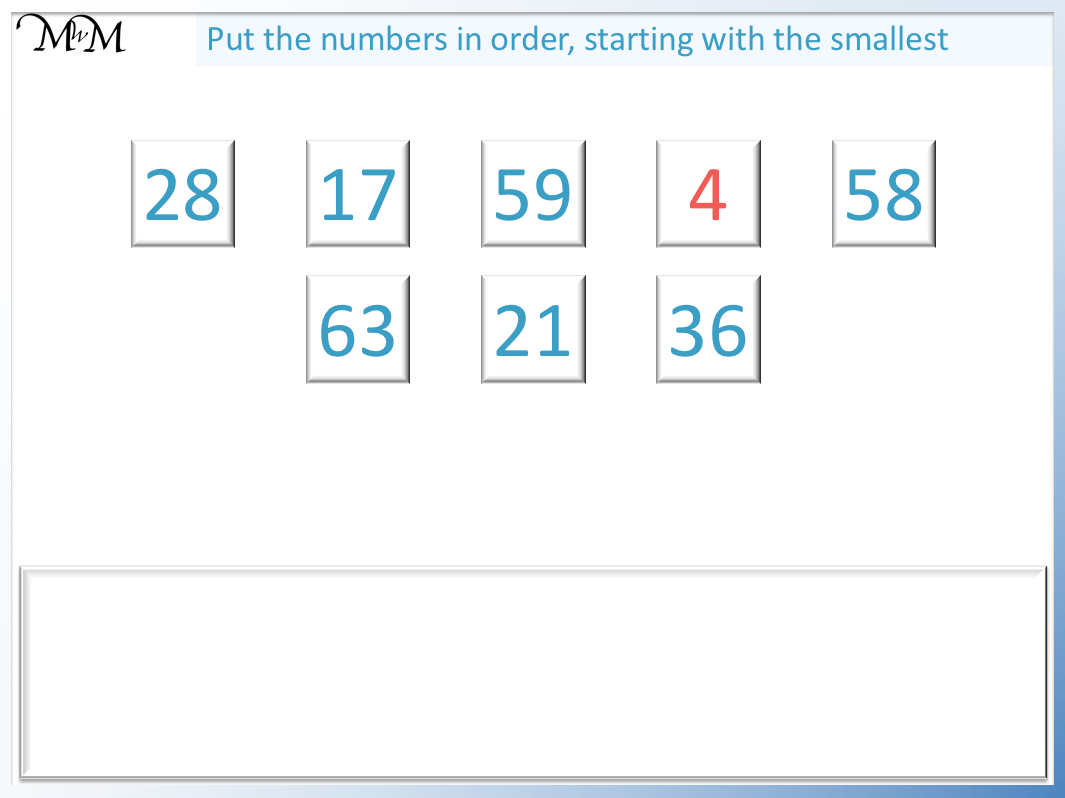This means that it is the only number smaller than ten and so, it is the smallest.

We could imagine the number ‘4’ as ’04’. The digit in the tens column is ‘0’.

We now look at the numbers with a tens digit of ‘1’.

17 is the only number in this list with a ‘1’ in the tens column. So it comes next.17 is the only number above that is larger than 10 but less than 20.

We have looked at numbers with a tens digit of ‘0’ and then ‘1’.

Next we look at the numbers in the list that have a tens digit of ‘2’.

21 and 28 both begin with a ‘2’.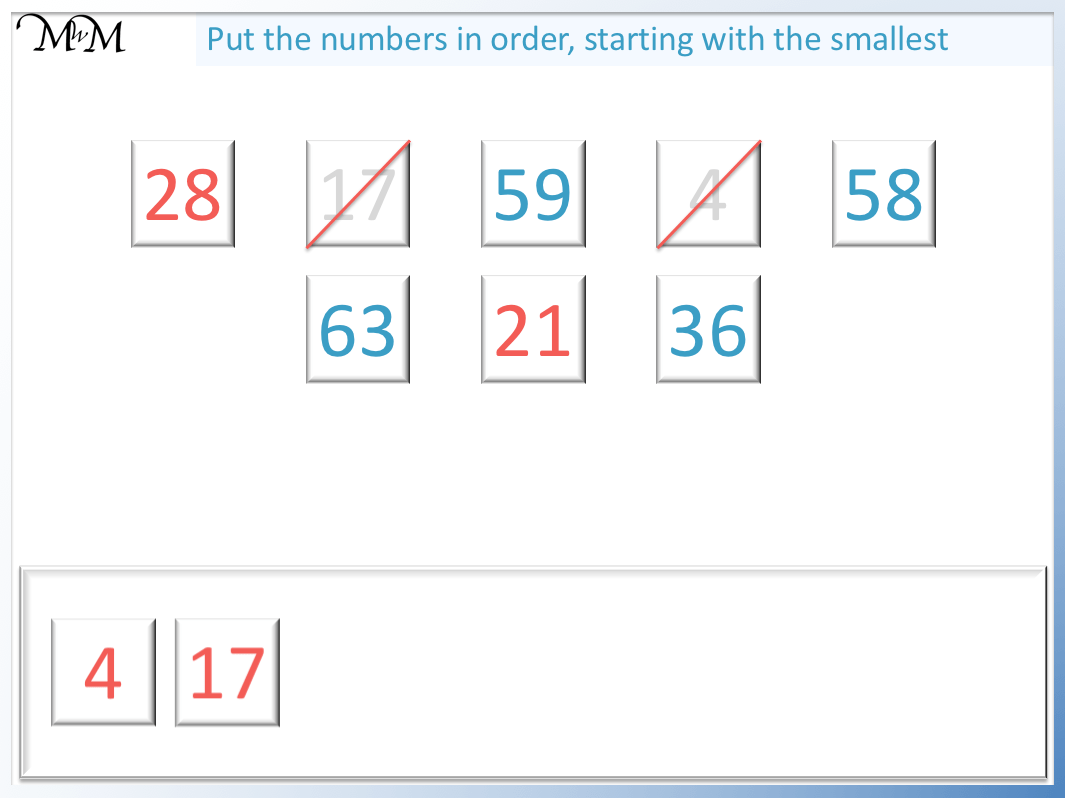Since both numbers both have the same tens digit, we move to the next digit on the right and compare these.

‘1’ is smaller than ‘8’ and so, 21 is smaller than 28.

Next we look at the numbers with a digit of ‘3’ in the tens column.

36 is the only number in this list with a tens digit of ‘3’. It is larger than 30 but less than 40.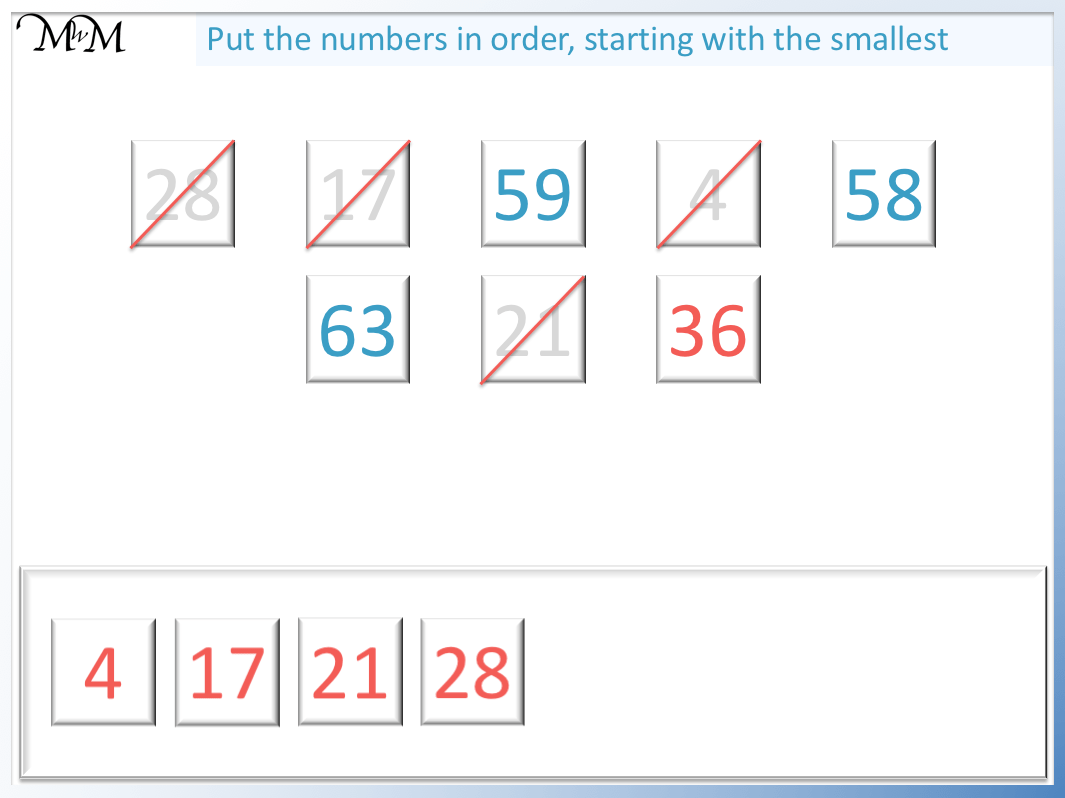We do not have any numbers that have a ‘4’ in the tens column.

This means that there are no numbers between 40 and 50.

We move on to look at the numbers that have a ‘5’ in the tens column: 58 and 59.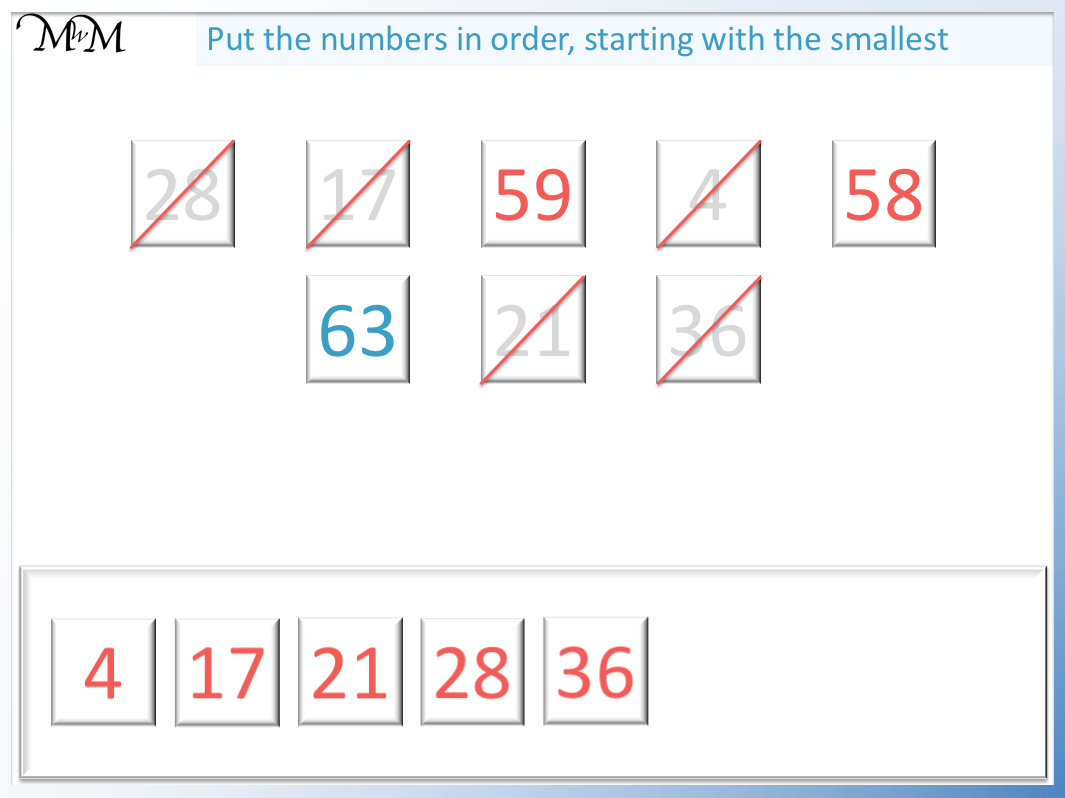Since both of these two numbers have a ‘5’ in their tens column, we look at the next digit along to compare their sizes.

‘8’ is smaller than ‘9’ and so, 58 is smaller than 59.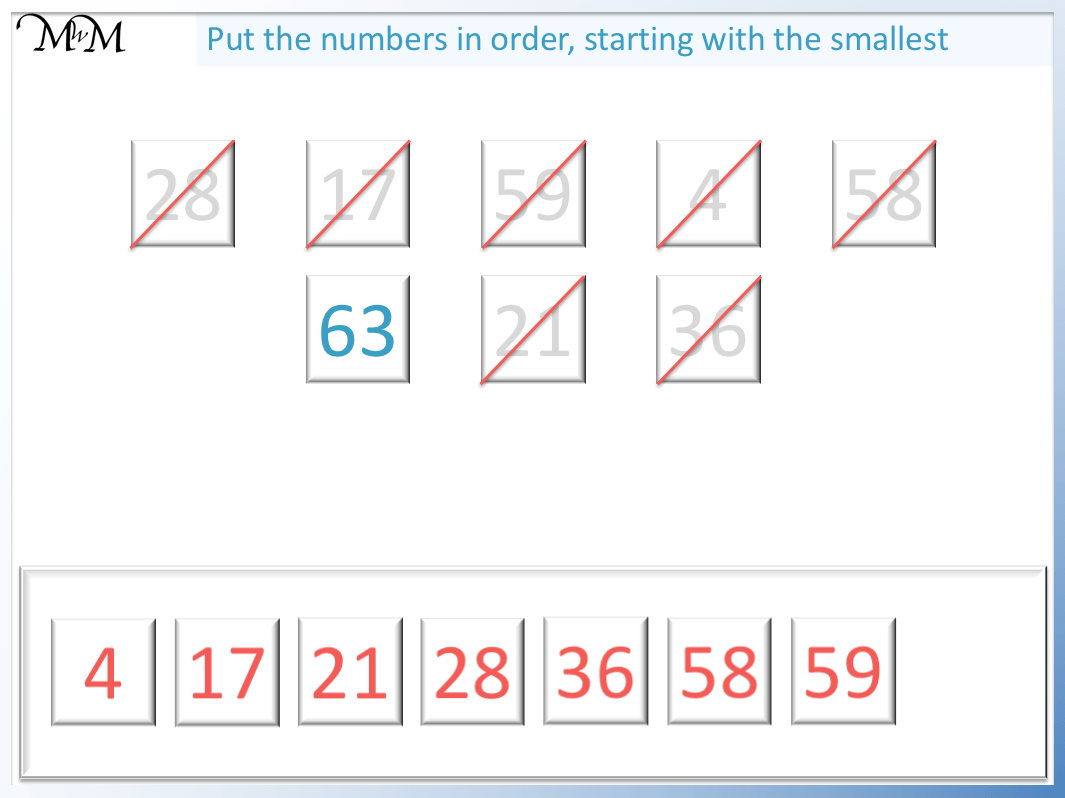The final number is 63 and it is the largest number because it has the largest digit in its tens column.

63 is the only number in our list that is greater than 60.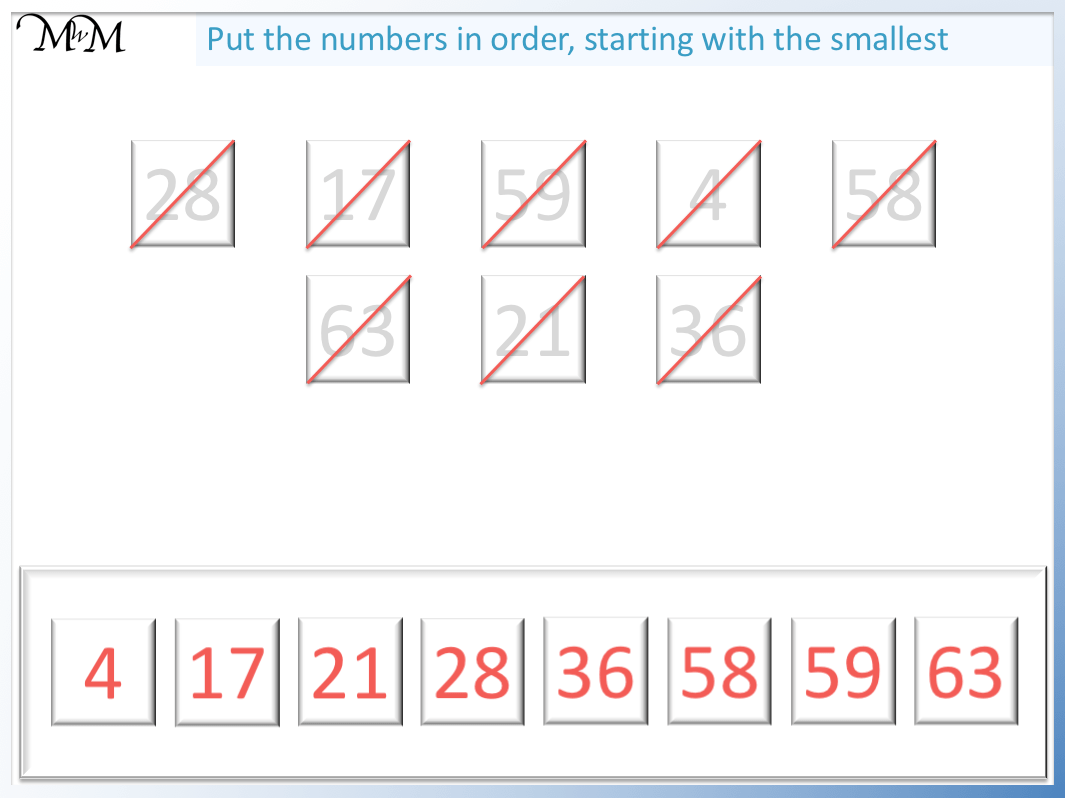Below is the animation which summarises the ordering of these two-digit numbers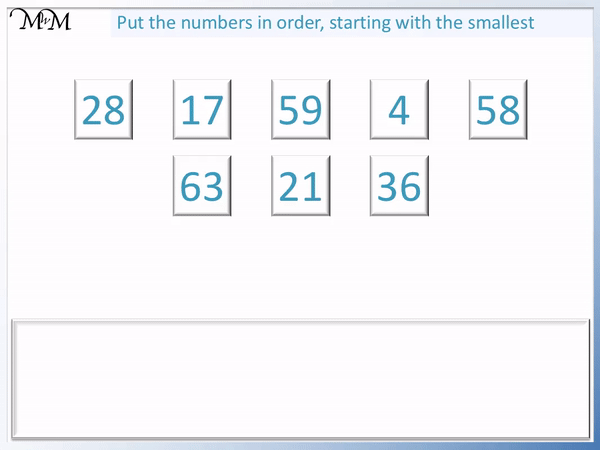Here is another example of ordering a list of numbers from least to greatest.We first consider the single-digit numbers which are less than 10.

We have 2 and 7.We can look at our list of digits and see that 2 is smaller than 7.

Next we look at numbers larger than 10 but less than 20.

We look at numbers with a tens digit of ‘1’.

These numbers are: 11, 12 and 14.These numbers all have a tens digit of ‘1’ and so we compare their units columns.

‘1’ is smaller than ‘2’ which is smaller than ‘4’.

Therefore 11 is smaller than 12 which is smaller than 14.

We have considered numbers with ‘0’ and ‘1’ tens.

Next we look at the numbers with a ‘2’ in the tens column.

We have 22, 25 and 29.These three numbers all have two tens.

We put them in order by considering their units column on the right.

‘2’ is smaller than ‘5’ which is smaller than ‘9’.

Therefore 22 is smaller than 25 which is smaller than 29.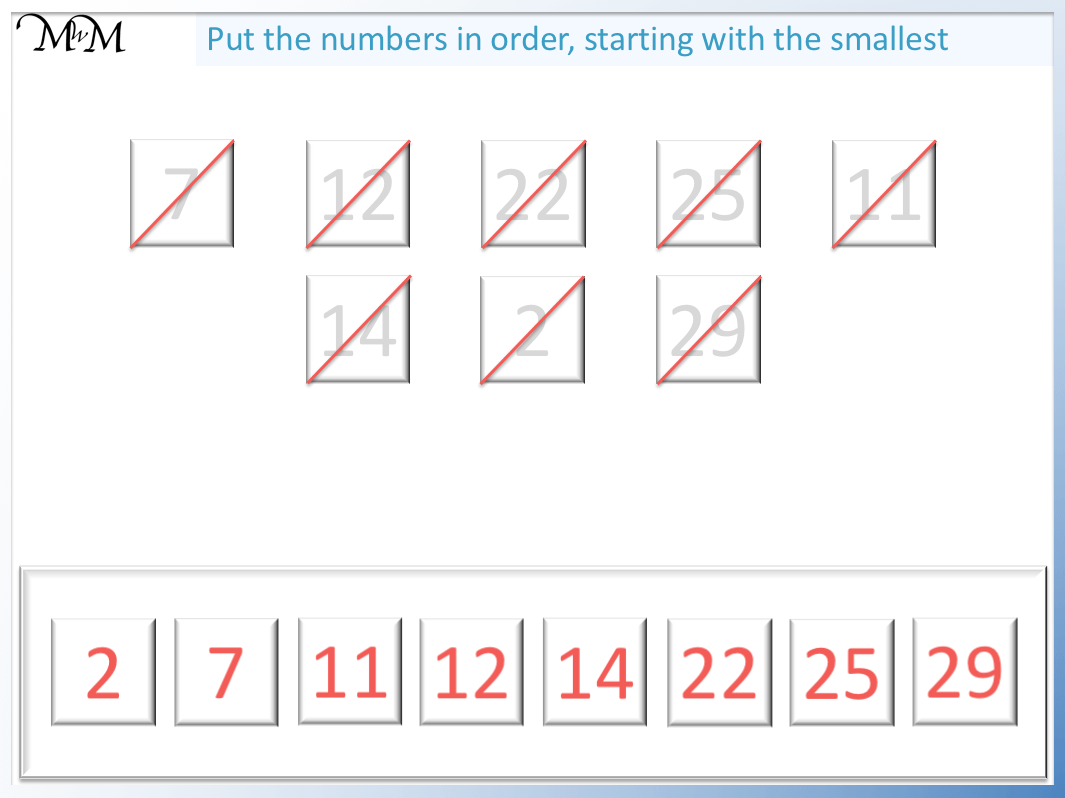Below is the full animation of putting this list of numbers in order from least to greatest.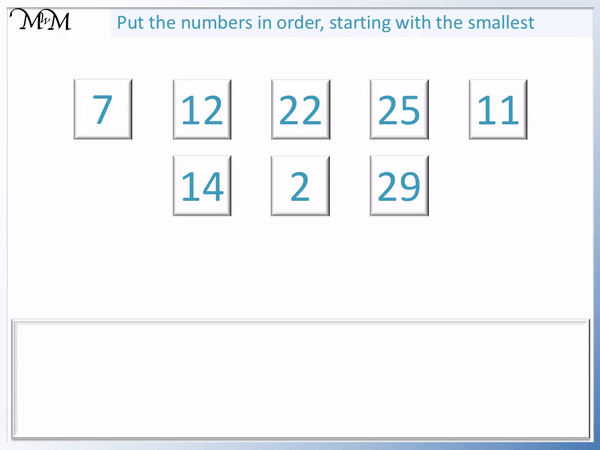Now try our lesson on Rounding to the Nearest Ten using a Number Line where we learn how to round numbers to the nearest ten.